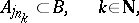# Brooks-Jewett theorem

Let $X$ be a topological group. A set function $m : \Sigma \rightarrow X$ is exhaustive (also called strongly bounded) if $\operatorname { lim } _ { n \rightarrow \infty } m ( E _ { n } ) = 0$ for each sequence $\{ E _ { n } \}$ of pairwise disjoint sets from the $\sigma$-algebra $\Sigma$ (cf. also Measure). A sequence $\{ m_i \}$ of set functions $m _ { i } : \Sigma \rightarrow X$, $i \in \mathbf{N}$, is uniformly exhaustive if $\operatorname { lim } _ { n \rightarrow \infty } m _ { i } ( E _ { n } ) = 0$ uniformly in $i$ for each sequence $\{ E _ { n } \}$ of pairwise disjoint sets from the $\sigma$-algebra $\Sigma$.

Being a generalization of the Nikodým convergence theorem, the Brooks–Jewett theorem [a1] says that for a pointwise-convergent sequence $\{ m _ { n } \}$ of finitely additive scalar and exhaustive set functions (strongly additive) defined on a $\sigma$-algebra $\Sigma$, i.e. such that $\operatorname { lim } _ { n \rightarrow \infty } m _ { n } ( E ) = m ( E )$, $E \in \Sigma$:

i) $m$ is an additive and exhaustive set function;

ii) $\{ m _ { n } \}$ is uniformly exhaustive.

There is a generalization of the Brooks–Jewett theorem for $k$-triangular set functions defined on algebras with some weak $\sigma$-conditions ($m : \Sigma \rightarrow [ 0 , \infty )$ is said to be $k$-triangular for $k \geq 1$ if $m ( \emptyset ) = 0$ and

\begin{equation*} m ( A ) - k m ( B ) \leq m ( A \bigcup B ) \leq m ( A ) + k m ( B ) \end{equation*}

whenever $A , B \in \Sigma$, $A \cap B = \emptyset$). The following definitions are often used [a2], [a6], [a5]:

SCP) An algebra $\mathcal{A}$ has the sequential completeness property if each disjoint sequence $\{ E _ { n } \}$ from $\mathcal{A}$ has a subsequence $\{ E _ { n_j} \}$ whose union is in $\mathcal{A}$.

SIP) An algebra $\mathcal{A}$ has the subsequentional interpolation property if for each subsequence $\{ A _ { j n } \}$ of each disjoint sequence $\{ A _ { j } \}$ from $\mathcal{A}$ there are a subsequence $\{ A _ { j n _ { k } } \}$ and a set $B \in \mathcal{A}$ such thatand $A _ { j } \cap B = \emptyset$ for $j \in \mathbf{N} \backslash \{ j _ { n_k } : k \in \mathbf{N} \}$.

According to [a5]: Let $\mathcal{A}$ satisfy SIP) and let $\{ m _ { n } \}$, $m _ { n } : {\cal A} \rightarrow [ 0 , + \infty )$, $n \in \mathbf N$, be a sequence of $k$-triangular exhaustive set functions. If the limit

\begin{equation*} \operatorname { lim } _ { n \rightarrow \infty } m _ { n } ( E ) = m _ { 0 } ( E ) \end{equation*}

exists for each $E \in \mathcal{A}$ and $m_0$ is exhaustive, then $\{ m _ { n } \} _ { n = 0 } ^ { \infty }$ is uniformly exhaustive and $m_0$ is $k$-triangular.

There are further generalizations of the Brooks–Jewett theorem, with respect to: the domain of the set functions (orthomodular lattices, $D$-posets); properties of the set functions; and the range (topological groups, uniform semi-groups, uniform spaces), [a2], [a4], [a5].

It is known that for additive set functions the Brooks–Jewett theorem is equivalent with the Nikodým convergence theorem, and even more with the Vitali–Hahn–Saks theorem [a3].